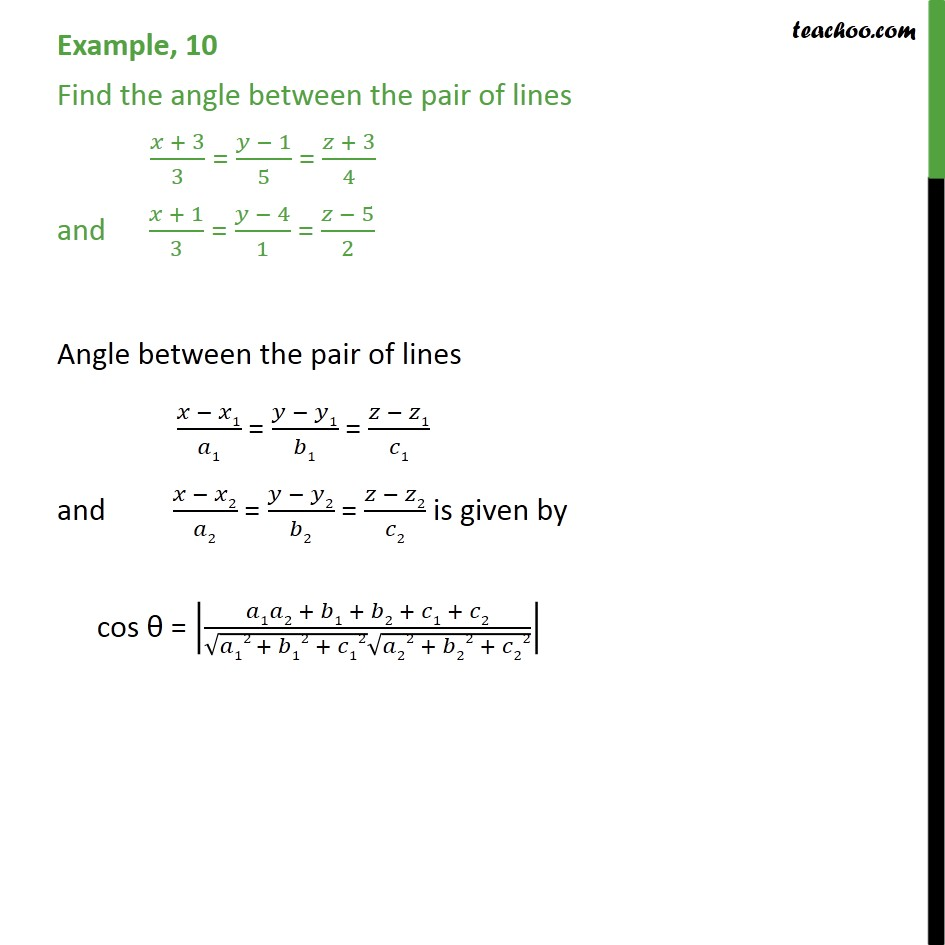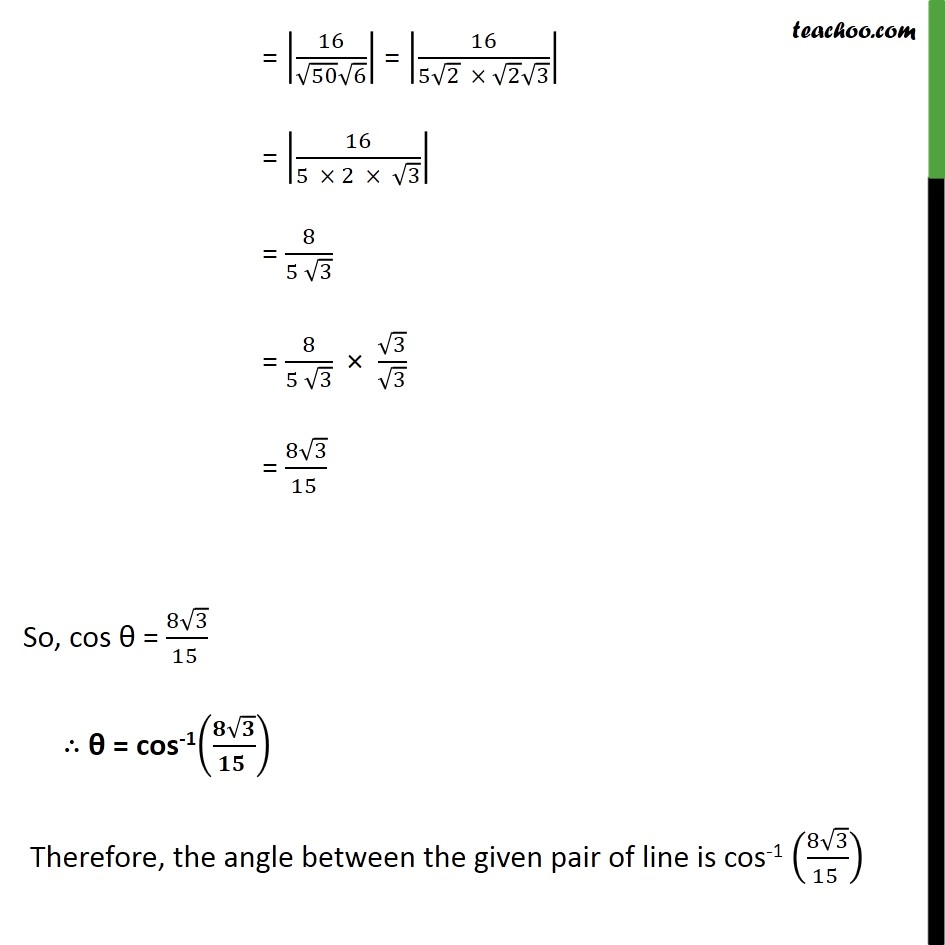1. Chapter 11 Class 12 Three Dimensional Geometry
2. Concept wise
3. Angle between two lines - Cartisian

Transcript

Example, 10 Find the angle between the pair of lines 𝑥 + 3﷮3﷯ = 𝑦 − 1﷮5﷯ = 𝑧 + 3﷮4﷯ and 𝑥 + 1﷮3﷯ = 𝑦 − 4﷮1﷯ = 𝑧 − 5﷮2﷯ Angle between the pair of lines 𝑥 − 𝑥1﷮𝑎1﷯ = 𝑦 − 𝑦1﷮𝑏1﷯ = 𝑧 − 𝑧1﷮𝑐1﷯ and 𝑥 − 𝑥2﷮𝑎2﷯ = 𝑦 − 𝑦2﷮𝑏2﷯ = 𝑧 − 𝑧2﷮𝑐2﷯ is given by cos θ = 𝑎1𝑎2 + 𝑏1 + 𝑏2 + 𝑐1 + 𝑐2﷮ ﷮𝑎12 + 𝑏12 + 𝑐12﷯ ﷮𝑎22 + 𝑏22 + 𝑐22﷯﷯﷯ = 16﷮ ﷮50﷯ ﷮6﷯﷯﷯ = 16﷮5 ﷮2﷯ × ﷮2﷯ ﷮3﷯﷯﷯ = 16﷮5 × 2 × ﷮3﷯﷯﷯ = 8﷮5 ﷮3﷯﷯ = 8﷮5 ﷮3﷯﷯ × ﷮3﷯﷮ ﷮3﷯﷯ = 8 ﷮3﷯﷮15 ﷯ So, cos θ = 8 ﷮3﷯﷮15 ﷯ ∴ θ = cos-1 𝟖 ﷮𝟑﷯﷮𝟏𝟓 ﷯﷯ Therefore, the angle between the given pair of line is cos-1 8 ﷮3﷯﷮15 ﷯﷯

Angle between two lines - Cartisian

About the AuthorDavneet Singh
Davneet Singh is a graduate from Indian Institute of Technology, Kanpur. He has been teaching from the past 9 years. He provides courses for Maths and Science at Teachoo.